# Atomic Orbitals and Nodes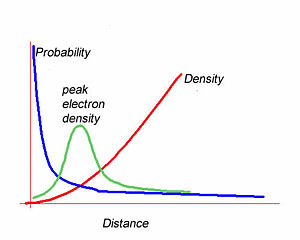Electron Probability, peak density and electron density as a function of distance from the nucleus.

## Contents

### Atomic Orbitals

Orbitals are important because they determine the distribution of electrons in molecules, which in turn determines the electronic and optical properties of materials. Atomic orbitals are wave functions that are solutions to the Schrödinger equation. This equation allows us to figure out the wave functions and associated energies in atomic orbitals.

The square of the wave function gives the probability of finding an electron at a certain point.

The integral of the wavefunction over a volume gives the enclosed electron density within that volume. The most likely position to find the 1s electron is at the nucleus.However the most likely radius is at some distance from the nucleus. The graph of wavefunction vs distance falls off exponentially as you move away from the nucleus. The electron density builds quadratically with distance from the nuclues. The peak electron density will be the product of the these two functions. This results in a curve with a density peak at a certain distance. Wavefunctions alone do not tell you the electron density.

### Orbital nodes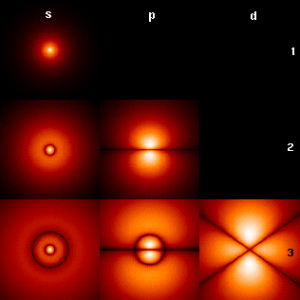Visualization of electron density as cross sections for orbitals and shells.

The p orbitals have orientations along the x, y and z axes. A node is a place where there is zero probability of finding an electron. A radial node has a spherical surface with zero probability. P orbitals have an angular node along axes. We usually indicate the sign of the wave function in drawings by shading the orbital as black and white, or blue and green.

1s: no node 2s: one radial node, 2p one angular node 3s: two radial nodes, 3p one radial node one angular node, 3d two angular nodes

The more nodes the higher the energy of the orbitals. The more the function varies spatially, the higher the energy.

### Core and valence electrons

Core electrons are very tightly bound tot he nucleus and spend most of their time very close to the nucleus. They are largely unaffected by the presence of nearby atoms.

Valence electrons are less tightly bound to the nucleus and are in the outermost "shell". These electrons are easily affected by the presence of other atoms and the ones that are critical for bonding between atoms.

According to the Aufbau principle we start adding electrons to the 2s orbital and then to the three 2p orbitals, each of which can have up to two electrons. The orbitals are filled from lowest energy to the highest. Here are the electron configuration for row two of the periodic table. The 1s orbitals are core while the two 2s and 2p orbitals are valence electrons. Neon has a full octet so it is non-reactive, ie a noble gas.$element$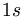$1s$$2s$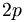$2p$
Li 1s2 2s1
Be 1s2 2s2
B 1s2 2s2 2p1
C 1s2 2s2 2p2
N 1s2 2s2 2p3
O 1s2 2s2 2p4
F 1s2 2s2 2p5
Ne 1s2 2s2 2p6

Here is the general rules for filling orbitals.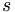$s$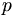$p$$d$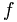$f$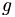$g$
1 1
2 2 3
3 4 5 7
4 6 8 10 13
5 9 11 14 17 21
6 12 15 18 22 26
7 16 19 23 27 31
8 20 24 28 32 36

### Bridging the Language Barrier

In working in an interdisciplinary research its easy to get confused with different terms for the same concepts as used by physicists and organic chemists. This table might help:

Physicist Speak Organic Chemist Speak
Bands Molecular Orbitals
Band Gap Excited State Energy
Excitons Excited States
High work function material Electron deficient - acceptor
Low work function material Electron rich -donor
!?&* stuff that messes up my vacuum chamber (fill in your favorite apparatus) Organic compound or polymer
Wavelength division mulitiplexer (fill you your favorite device system) Device thingy